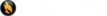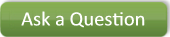Home About Engineering Witness & Problem Solving Computer Programs Projects Studies Contact

# Flame Temperature Calculation Procedure Using the Calculated Composition of the Combustion Products and the Energy Inputs. Example for Standard Natural Gas Burned in a Furnace

[ back to full computer program list ]

Knowing the fuel consumption (Btu/hour-HHV) and the Percent Excess Air that is needed to support the combustion process an engineer can then calculate the Mass flow (lbs/hr) of combustion gases that are formed. If we also know the properties on the incoming combustion air it is then possible to calculate the flame temperature. Once this data is identified the CPGASH56 Program can be used to calculate the flame temperature.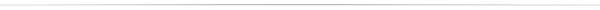## Example #1 - Flame Temperature Calculation Procedure This Example Involves a Low Efficiency Induced Natural Gas Furnace

1. Fuel: Natural Gas
HHV (Btu/lb) = 22,708.49
HHV (Btu/ft3) = 1040
Consumption = 100,000 Btu/hr
Mass Flow = 4.4036 lb/hr

2. Combustion Air
Dry Bulb = 70 F
Relative Humidity = 35 %
10 % Excess Air
ACFM = 17.8468
Mass Flow = 79.9282 lb/hr
Total Energy Flow = 1,194.31 Btu/hr

3. Total Furnace Input Energy = 101,194.31 Btu/hr

4. Combustion Analysis Gases Formed
a. CO2 = 11.7888 lb/hr
b. N2 = 61.0971 lb/hr
c. O2 = 1.6725 lb/hr
d. SO2 = 0.0001 lb/hr
e. SO3 = 0.0000 lb/hr
f. Argon = 0.0934 lb/hr
g. Water Vapor = 9.6800 lb/hr
h. Total Flue Gas= 84.3319 lb/hr

5. Calculation
Using the above combustion gas Mass Flows the engineer by trial and error can input different assumed flame temperatures until the Total Energy for that Mass Flow is at 101,194.31 Btu/hour. The result of that computer analysis is the CPGAS.txt document below.

### CPGAS.txt

Complete Energy Analysis for a Lower Efficiency Induced Draft Natural Gas Furnace (PDF)

### Alternative Approach Used to Determine the Flame Temperature for Natural Gas Combustion

There is another approach that can be used to determine the flame temperature from a natural gas furnace. The attached diagram uses the Percent Excess Air and the Percent Available Heat (this is the percentage of input energy that is delivered).

1. Percent Excess Air: 0 % to 1000 % (assumes 60 F combustion air)

2. Percent Available Heat =
Total Heat Delivered (Btu/hour) x 100
Total Heat Into The Furnace (Btu/hr)

For this specific case you enter the diagram with the following data:

Percent Excess Air = 10.00 %

Percent Available Heat = 0.00 %

There is no available heat (heat delivered to the home) because we are looking at the burner which is before the heat exchanger that captures the heat for the home.

Chart Value For Flame Temperature = 3310 F

Flue Gas Temperature Illustration (PDF)

[ back to full computer program list ]      [ back to top ]

## Articles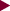Consulting Engineering Condensation Problem Solving Dehumidification Forensic Investigations & Building Diagnostics Air Contaminant Problem Solving & Indoor Air Quality Fuel Properties & Combustion Analysis Dryer & Hood Engineering Electricity Generation & Estimation of Levelized Cost Energy Analysis & Heat Recovery General Engineering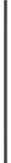Computer Program Examples Building Water Vapor Transmission & Condensation Ductwork Heat Loss or Heat Gain & Condensation Insulated Pipe or Flat Surface Heat Loss or Gain Psychrometrics of Air & Water Vapor Mixtures Properties of Compressed Air Belt Drive Loss Fan Performance Analysis     [ More.. ](920) 465-3548 PO Box 8695 Green Bay, WI 54308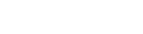Copyright © 2011 Acker & Associates Website By